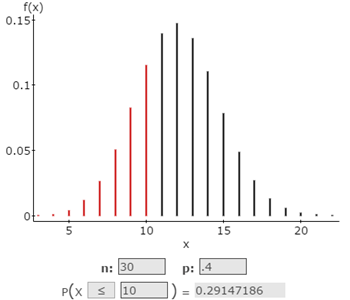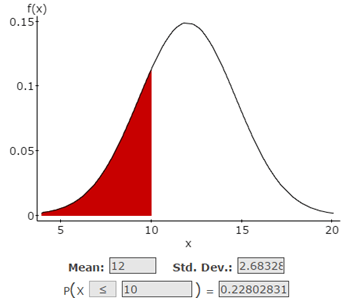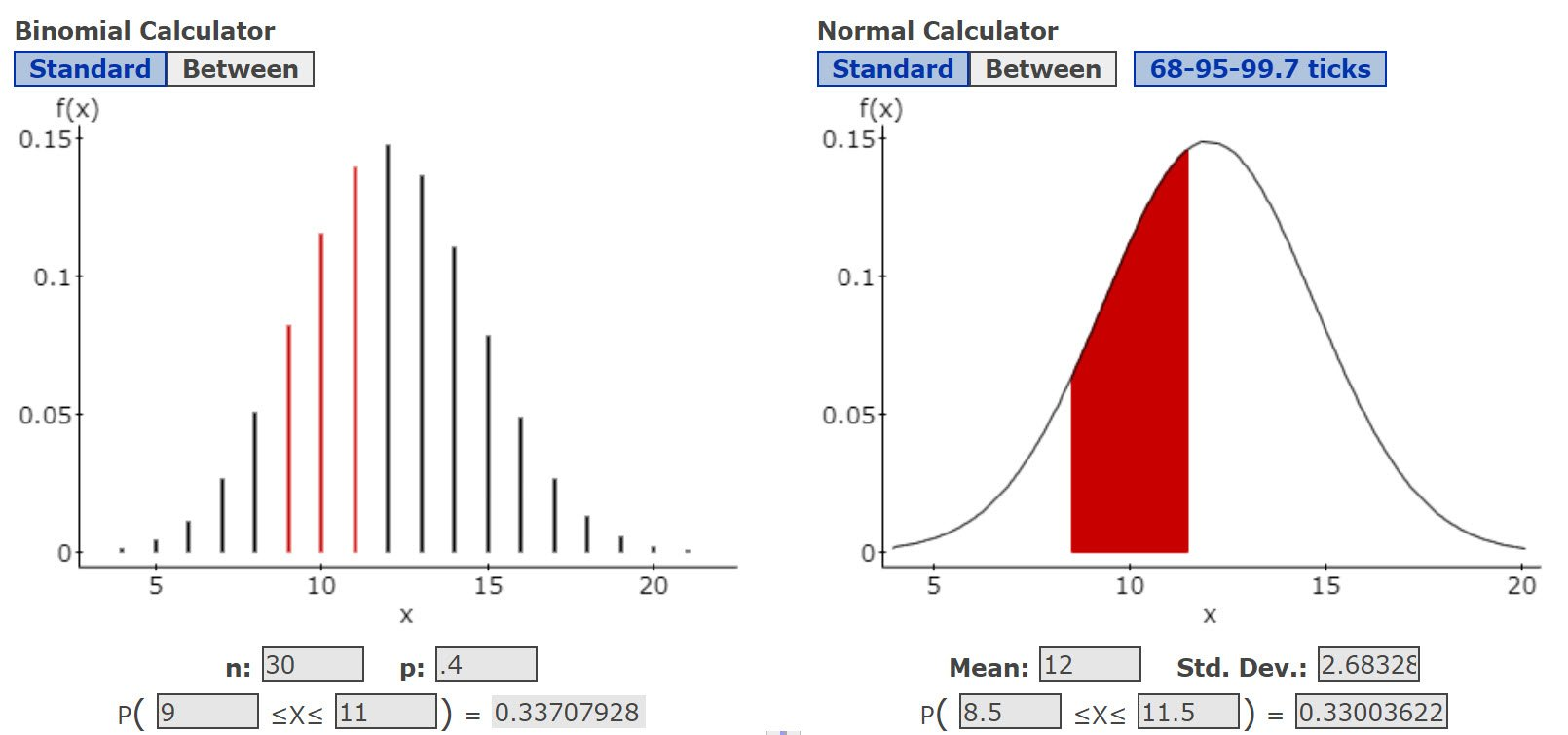# Continuity Correction – Filling the cracks in the Normal Approximation to the Binomial

When we approximate a discrete distribution, such as the binomial, by a continuous distribution, such as the normal, we need to make adjustments so “things don’t fall in the cracks.”

From the Central Limit Theorem, we know that a sample distribution from a population, even a non-normal one, becomes normal if the sample size is large enough. Though we often think of “large enough” to be 30, we need to be careful with binomial distributions.

For binomial distributions, which are defined by n and the proportion/probability p, both n times p and n times q, which is (1-p), need to be greater than 5. Once we confirm that both are greater than 5, we need to apply the continuity correction before we are able to use the normal curve to find our answers.

Remember that a binomial distribution is a discrete distribution and can only take integers as values. The normal distribution can take any real number, which means fractions or decimals. Thus, the binomial has “cracks” while the normal does not.

Here is a graph of a binomial distribution for n = 30 and p = .4. This was made using the StatCrunch™ binomial calculator and I set it to show the probability of x being 10 or less (≤). The red bars indicate the discrete values of 1 through 10 that are included in this range. The probability of getting a 10 or less, P(x ≤ 10), is 0.291, rounding to three decimals.Recalling that the mean of a binomial distribution is just n times p, we get μ = 12. The standard deviation of that same distribution is the square root of npq which is 2.683282.

I like to keep a lot of decimal places so I can round with more confidence at the last step.

Recall also that a normal distribution is defined by its mean and standard deviation.

Here is the StatCrunch™ normal calculator for that same mean, 12, and standard deviation, 2.683282, and for P(x ≤ 10):
Hint: remember the math operator points to the tail of the normal curve, ≤ points to the left!But wait! The probability approximated by the normal curve is a lot smaller, 0.228.  That is not very close, is it?

The mistake we made is that we did not account for the gap between 10 and 11 on the binomial distribution.

If we re-run the normal calculator with P(x ≤ 11), which would cover the entire gap between 10 and 11, we get a probability of 0.355, which is too large.

Thinking about this, it seems using P(x ≤ 10) is missing some of the area under the normal curve between 10 and 11, while using P(x ≤ 11) includes too much area. We need to make sure we include all of the integer 10 from the binomial distribution, but none of the integer 11. How do we make the two distribution curves more equivalent?

The way we fix this is to split the gap between the integers 10 and 11 into two parts, and assign half to 10, to make it end at 10.5; and the other half to 11, which would make it start at 10.5. That way, the gap is accounted for, or “corrected for continuity” between 10 and 11.So, running the normal calculator again with P(x ≤ 10.5), we get a probability of 0.288, just 1 percent smaller than the probability of 0.291 we found using the binomial.

Setting up for the Continuity Correction

To use the normal approximation, we need to remember that the discrete values of the binomial must become wide enough to cover all the gaps. You can think of it as each integer now has a -0.5 and a +0.5 band around it. Number 1 covers 0.5 to 1.5; 2 is now 1.5 to 2.5; 3 is 2.5 to 3.5, and so on.

Next, when you read a problem asking you to use the normal approximation for the binomial, look for keywords and phrases and then check this Words to Math Operators table:For example, if the problem states: “Find the probability that at least 10 people say Yes,” that would mean you need to choose the ≥ math operator. Then your statement would be to find P(x ≥ 10)

You will probably not run into a question requiring a not equal (≠) operator. That is a good thing because the StatCrunch calculators do not have a tool to solve for a ≠. If you do run into one, recall that the probability for x ≠ to a value, n, is just 1 – the probability of x = n.

Once you find the right math operator, use this table to set up the continuity correction to use in the normal approximation:Notice the pattern that cases where the = math operator is seen (<=, =, >=), the continuity corrected value to use includes c.

• Here are the StatCrunch™ calculators for the first example, P(x = 10). Note, we use the “Between” part of the Normal Calculator. You can see the red bar representing 9.5 to 10.5 on the Normal graph.• This is the second example, P(x > 10). The approximation for the probability of getting integers 11 through 30 starts at 10.5 and goes to the right tail.
• The third example, P(x ≤ 10), we did initially. The probability (area) from the normal approximation of getting integers 1 through 10 starts at the left tail and goes through 10.5.
• The fourth example, P(x < 10), we get the following. The normal approximation of getting integers 1 through 9 starts at the left tail and goes through 9.5.
• The 5th example, P(x ≥ 10). The normal approximation of getting integers 10 through 30 starts at 9.5 and goes to the right tail.
• And the last example, P(9 < x < 11).  Here, we use the ‘between” version of the calculators to find the normal approximation closely matched the binomial probability.Hope this helps! If you prefer to work with Excel, check out this calculator: https://www.drdawnwright.com/?p=17877

This site uses Akismet to reduce spam. Learn how your comment data is processed.